# Sequences with Perfect Periodic Auto and Cross Correlation Properties

Sequences with Perfect Periodic Auto and Cross Correlation Properties
Department of Electronics and Communication Engineering, Mahatma Gandhi Institute of Technology, Hyderabad 500075, India

Corresponding Author Email:
tdbhatt_ece@mgit.ac.in
Page:
477-484
|
DOI:
https://doi.org/10.18280/ts.370315
1 March 2020
|
Accepted:
26 May 2020
|
Published:
30 June 2020
| Citation

OPEN ACCESS

Abstract:

Perfect binary or ternary sequences exhibit an impulse-like Periodic Autocorrelation Function (PACF). Signals with perfect periodic autocorrelation properties are useful in many applications in communications and radar fields. The additional complexity in the design of radar signals is to take care of the cross-correlation properties when multiple radars are sharing the same frequency band. This paper proposes the waveform design approach related to the multi-radar network, when radars are employing coherent pulse train or CW waveforms. Such practical scenario demands the design of waveforms which have perfect autocorrelation and cross-correlation properties. Construction procedure, correlation properties and detection performance of such sequences are presented in this paper.

Keywords:

periodic autocorrelation, cross-correlation, periodic ambiguity function, zero-correlation zone (ZCZ), synthesized sequences

1. Introduction

Discrete-time signals or sequences with impulse like autocorrelation functions have many applications in measurements, communications and pulse compression radar systems. Pulse compression is a technique, which is commonly used by radar, sonar and echography to increase the Signal-to-Noise Ratio (SNR) and range resolution. To achieve this, long duration modulated pulses are transmitted and received signal is correlated with transmitted pulse. Most of the research related to radar signals are centered around the design of waveforms for active radar system that uses Aperiodic Autocorrelation Function (AACF) . In general, it is not possible to find sequences with an ideal AACF . However, CW signals with periodic modulations can yield an ideal autocorrelation and are extensively used for radar applications. The properties and aptness of such signals are studied in [1, 3, 4]. A binary sequence, which has Perfect Periodic Auto-correlation Function (PPACF) of length 4 is [1 1 1 -1]. Furthermore, when a network of multiple radar sensors is used, the sequences with ideal periodic auto and cross-correlation properties are the optimum choice [5-7]. In a network of radar sensors, the interference among different radars can be minimized efficiently when sequences with good cross-correlation property are designed .

This paper is focused on the construction of binary sequences with higher energy efficiency that display perfect cross-correlation properties in addition to the ideal autocorrelation within the Zero Correlation Zone (ZCZ). Results presented here show that these sequences are optimal in Radar Sensor Network (RSN) and dense target environment, particularly when radars are employing coherent pulse train or CW waveforms. These sequences are termed as “synthesized sequences”.

The remainder of the paper is arranged as follows. Section 2 discusses properties of periodic sequences. Section 3 deals with the design of sequences with perfect periodic autocorrelation. Section 4 explains construction of synthesized sequences with perfect periodic auto and cross correlation properties. Results and discussions are provided in section 5. Finally, Section 6 provides with the conclusions of the study.

2. Properties of Periodic Sequences

Let si(n) be a real sequence of length N and the periodic repetition of si(n) with period N gives the periodic sequence ŝi(n). The autocorrelation and cross-correlation functions of such periodic sequences can be given by:

$R_{i i}(\tau)=\sum_{n=0}^{N-1} \hat{s}_{i}(n) \hat{s}_{i}^{*}(n+\tau) 0 \leq \tau<N$      (1)

$R_{i j}(\tau)=\sum_{n=0}^{N-1} \hat{s}_{i}(n) \hat{s}_{j}^{*}(n+\tau) 0 \leq \tau<N$      (2)

$R_{i i}(\tau)=\left\{\begin{array}{c}E, \text { for } \tau=0 \\ 0, \text { for } \tau \neq 0\end{array} 0 \leq \tau<N\right.$     (3)

where, ŝi(n+τ)=si((n+τ) mod N), and τ=mtb is time delay and tb is duration of single bit. Eq. (1) is representing the autocorrelation function and Eq. (2) gives the cross-correlation function of such periodic sequences.

The energy ‘E’ associated with the sequence is given by

$E=\sum_{n=0}^{N-1} s_{i}^{2}(n)$     (4)

Luke  proposed the methods to synthesize sequences having perfect periodic autocorrelation functions and good energy efficiency. These sequences are synthesized by modifying binary sequences, ternary sequences, M-sequences, Legendre sequences, product of two synthesized sequences and using computer search. The sequences are synthesized by taking advantage of one of the important properties that the magnitude of the spectrum of perfect sequences is constant and it is given by:

$\tilde{\mathrm{S}}_{i}(\mathrm{k})=\sum_{n=0}^{N-1} \hat{\mathrm{s}}_{i}(n) \exp (-j 2 \pi n k / N) 0 \leq k<N$     (5)

By setting ‘k’ to zero, Eq. (5) becomes:

$\left|\tilde{S}_{i}(0)\right|=\left|\sum_{n=0}^{N-1} s_{i}(n)\right|=\sqrt{E}$     (6)

In his work , Luke tabulated the perfect sequences up to length 60, which have good energy efficiencies. The efficiency η of a sequence can be given by Eq. (7). The efficiency of these sequences is degraded due to their non-uniform amplitude. Therefore, in case of taking product of two non-uniform periodic sequences, the efficiency further decreases to η=η1η2, where η1 and η2 are efficiencies of two sequences. Formula for calculating η is:

$\eta=\sum_{n=0}^{N-1} \frac{s^{2}(n)}{\left|s^{2}(n)\right|_{\max }}$     (7)

2.1 Periodic ambiguity function

The Periodic Ambiguity Function (PAF) is another tool, which plays an important role for the design of radar signals and similar to the ambiguity function used for finite duration signals. Ambiguity function is the study of the matched filter output, when the received signal is exactly of the same finite duration as the transmitted signal except shifted in time (delay τ) and Doppler (υ) . The similar concept can be extended to the periodic signals by considering finite due to finite dwell time [8-11]. Let the dwell time of the signal is PT, where T is modulation period and P˃1. On receive the return signal is correlated with reference signal in matched filter for N (an integer) periods of transmitted signal with M˂P, which is shown in Figure 1.

## 1.png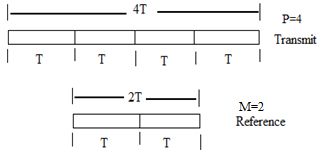Figure 1. Relative time durations of transmitted and reference signals

If s(t) is the transmitted signal than it obeys:

s(t)=s(t+nT)      (8)

where, n=±1, ±2, ±3….

During the interval τ≤(P-M)T, the correlator output response in delay-Doppler plane is periodic ambiguity function and can be given as:

\begin{aligned}\left|\chi_{N T}(\tau, v)\right|=\frac{1}{N T} |& \int_{0}^{N T} s\left(t+\frac{\tau}{2}\right) s^{*}(t\\ &\left.-\frac{\tau}{2}\right) \exp (j 2 \pi v t) d t| \end{aligned}      (9)

where, τ is delay constant and υ is Doppler shift.

The relationship between the PAF of N periods and single-period ambiguity function can be given by:

$\left|\chi_{N T}(\tau, v)\right|=\left|\chi_{T}(\tau, v)\right| \mid \frac{\sin (\pi v N T)}{N \sin (\pi v T)}$      (10)

where,

$\left|\chi_{T}(\tau, v)\right|=\left|\frac{1}{T} \int_{0}^{T} s(t+\tau / 2) s^{*}(t-\tau / 2) \exp (j 2 \pi v t) d t\right|$  is the ambiguity function of single-period signal. This equation gives an important result of PAF in terms of ambiguity function of a single period multiplied by function similar to sampling function of N and T [1, 9].

2.2 Cyclic shift property of periodic sequences

Let us consider a sequence Xn=[x1, x2, x3,…, xN] of length N. any of its shifted sequence after ‘s’ cyclic shifts X(n+s)=[xs, xs+1, …, xNx1, x2, x3,… xs-1] where 1≤sN. If we consider, X5=[0 0 0 1 1] than after one shift X5+1=[1 0 0 0 1]. Sequence achieved after five shifts is an original sequence. Therefore, the periodic autocorrelation property of the shifted sequence is same as the original sequence, since the periodic autocorrelation property is invariant to cyclic shifts. However, the aperiodic autocorrelation function of cyclically shifted sequence may be different from the original one. Next section explains construction of binary sequences which have perfect periodic autocorrelation property.

3. Sequences with Perfect Periodic Autocorrelation

Two important sequences, M-sequences and Legendre sequences are considered here for the design of synthesized binary sequences. M-sequences and Legendre sequences exhibit lowest PACF equal to |Rii(τ≠0)=1|. The Ipatov code  is also a code pair which exhibits perfect periodic autocorrelation (the cross correlation of the code pair) and minimal mismatch loss. But the construction method of reference code for Ipatov pair is complicated. M-sequences and Legendre sequences having code length N produce periodic autocorrelation of peak value equal to N and uniform sidelobes of value 1. Figure 2 shows the periodic autocorrelation function of M-sequence of code length N=7. The PACF clearly show that the magnitude of sidelobes other than peak is ‘1’ which is constant. Levanon  and Jahangir  demonstrated the ideal periodic correlation properties of M-sequences and Legendre sequences by taking the cross-correlation between the unipolar version {1, 0} of transmitted signal and the bipolar reference signal {±1}. It is shown that this method is advantageous in context of Non-coherent Pulse Compression (NCPC) radar. These sequences are also optimized to achieve perfect auto and cross correlation properties and referred as optimized Punctured ZCZ Sequence-pair Sets (PZCZSPS), proposed by Lei and Liang [5, 15, 16]. The periodic cross-correlation property of punctured M-sequence for N=7 is shown in Figure 3 where all off-peak sidelobes are zero. To achieve zero sidelobes, the number of ‘1’s must be larger than the number of ‘0’s by one element in a given M-sequence. It can be observed from Figure 3 that the peak lobe value is 4 because the number of ‘1’s in the transmitted signal are 4 and ‘0’s are 3. The perfect periodic cross-correlation property is achieved at the cost of high energy loss. The energy efficiency of unipolar M-sequence (N=7) is nearly 57% and such signals are preferred in non-coherent processing [13, 14].

## 2.png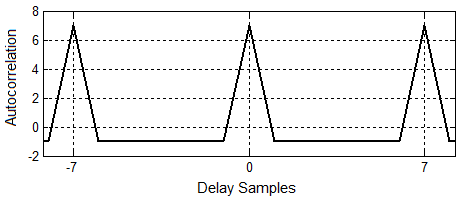Figure 2. Periodic autocorrelation function of M-sequence N=7

## 3.png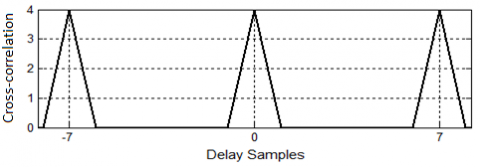Transmitted sig=[1 1 1 0 1 0 0]; Reference sig=[1 1 1 -1 1 -1 -1]

Figure 3. Periodic cross-correlation of M-sequence N=7

For coherent processing, the reference signal must be same as the transmitted signal. Therefore, there is a need to design synthesized binary sequences which have perfect periodic autocorrelation property with higher energy efficiency so that these sequences can be used in high resolution radar applications.

To enhance the detection capability of a radar system, the energy associated with reflected echo must be large which is directly proportional to the transmitted energy of the signal. On the other hand, range resolution depends upon pulse compression, which is equal to N, where N is length of the sequence. Therefore, it is significant to discuss the construction of the sequences which have higher energy efficiency and large sequence length. Luke  presents such sequences only up to lengths 60 and the maximum energy efficiency of these sequences is less than or equal to 82% except for a ternary sequence of length 57. In this paper perfect amplitude-symmetric binary sequences of high energy efficiency above lengths 60 are investigated and listed in Table 1. These sequences are derived either from M-sequences or Legendre sequences which can be written in the form of (N+1)/2 positive ‘1’s and (N-1)/2 negative ‘1’s. Another major advantage of such binary sequences is as the length of the sequence increases, the energy efficiency also increases and approaches 1 as N approaches infinity . Table 1 shows the synthesized binary sequences which have energy efficiency above 82%. This table includes sequences form N=63 to N=199, which satisfy the condition (N+1)/2 positive and (N-1)/2 negative bits in the sequences and all are prime numbers except 63.

In all synthesized binary sequences the value of ‘a’ is calculated by using Eq. (11), where N is an odd integer.

$a=\frac{-1}{1+\frac{2}{\sqrt{N+1}}}$     (11)

Though this paper includes synthesized binary sequences only up to length 199, but such sequences can be designed for any sequence length N, where N≡3(mod4), and N is prime number. Next section explains the design of larger length sequences which are not prime, by using product of two sequences.

Table 1. Synthesized binary sequences with energy efficiency η>82%

 N η (%) Sequence of good energy efficiency 63 82.3 a  a  a  a  a  1 a  a  a  a 1 1 a  a  a 1 a  1  a  a  1  1  1  1  a  1  a  a  a  1 1 1  a  a  1  a  a  1  a  1  1  a  1 1  1  a  1  1  a   a  1  1  a  1  a  1  a  1  1 1 1 1 1 (a=-0.8) 67 82.7 1  a 1 1 a 1 a 1  1  a   a  1  1  1  a  a  a  a  1 a 1 a  a  a  a  a  a  1 1 a 1 1 1 a  1  a a  a  1  a  a  1 1 1  1 1 1 a 1 a 1 1  1 1 a  a  a  1 1  a  a  1 a 1 a  a 1  ( a=-0.805) 71 83 1 a a a a  a  a 1 a  a   a  1  a 1 1 a  a  1 a  a  a 1 1 1 a  a  1 a 1 a  a  1 a  1 1 1 a  a  a  1  a  1 1  a  1  a 1 a  a  a  1 1  1 a  1 1  a  a 1 a  1  1 1 a  1 1  1 1 1 1 (a=-0.81) 79 83.6 1 a a 1 a  a 1 1 a  a  a  a  1  a  1 1  a  1 a  a  a  a  a  a  1  a  a  1  1 1 1 a  a 1 1 1 a  1 a 1  a  1  a  1  a   a  a  1  1  a  a   a   a  1 1 a  1  1 1  1  1  1 a 1  a  a 1  a 1 1 1 1 a  a  1 1 a 1 1   ( a=-0.817) 83 83.9 1 a  1  a  a  1  1  a  1  a   a   a  a  1  1  1  a  a  1  1  1  a  1  a  1  a  a  a  a  a  a  a  1  a  1     1  a  a  a  1  a  a  1  1  a  1  1  1  a  a  1  a  1  1  1  1  1  1  1  a  1  a  1  a  a  a  1  1  a  a   a  1  1  1  1  a  1  a  a  1  1  a  1  (a=-0.821) 103 85.1 1  a  a  1  a  1  1  a  a  a  1  1  1  a  a  a  a  a  a  a  1  1  1 a  1  a  a  1  a  a  a  1  a  a  a  1   a  1  a  1  1  a  1  1  1  1  a  1  1  a  a  1  a  1  1  a  a  1  a  a  a   a  1  a  a  1  a  1  a  1  1    1  a  1  1  1  a  1  1  a  1  a  a  a  1  1  1  1  1  1  1 a  a  a 1  1  1 a  a 1 a 1  1  (a=-0.836) 107 85.3 1 a 1 a  a 1  1  1  1 a  a  a  a  a  a 1 a 1  a 1  1  1  a  1 a 1 a 1  a  a  1 1 a  a  a  a  a  1  a  a  a  a  1  a  1  1 a  a  a 1  1 a  a  1  1 a  a  1  1  1  a  a  1  a  1  1  1  1 a  1  1  1  1  1  a  a  1  1  a  1 a 1 a  1  a  a  a  1  a  a  1  a  1  1  1  1  1  1  a  a  a  a  1  1 a 1  (a=-0.839) 127 86.2 1  a  a  1  a  1  1  1  a  a  1  a  1  a  1  a  a  a  a   a  1  a  a  1  1  a  a  1  1  1  a  a  a  1  a   a     a  a  a  1  1  a  a  1  a  1  1  a  1  a  a  1  a  1  1  1 1  1  1  1  a  a  a  1  a  1  1  1  a  a  a  a  a   a  a  1  a  1  1  a  1  a  a  1  a  1  1  a  a  1  1  1  1  1  a  1  1  1  a  a  a  1  1  a  a  1  1  a     1  1  1  1  1  a  1  a  1  a  1  1  a  a  a  1  a  1  1   (a=-0.85) 131 86.4 1 a  1  a  a  a  1  a  1  a  1  a  a  a  1  a a  1  1  1  a  a  1  1  1  a  1 a  a  1  1  1  1  a  a  a  a   1  a  a  1  a  1  a  a  a  a  1  a  a  1  1  a  a  1  a  1  1  a  a  a  a  a  a  a  a  1  1  1  1  1  1  1    1  a  a  1  a  1  1  a  a  1  1  a  1  1  1  1  a  1  a  1  1  a  1  1  1  1  a  a  a  a  1  1  a  1  a  a    a  1  1  a  a  a  1  1  a  1  1  1  a  1  a  1  a  1  1  1  a  1   (a=-0.852) 139 86.6 1  a  1 1  a  a  a  a  1  a  1  a  1  a  1 1  a 1  1  1  a  1  1  1  a  a  1  1  a  a  a  a  1  1  a  a  a    a  a  1  1  a  a  1  a  a  a  a  1  a  1  a  a  1  a  a  1  a  1  1  1  1  1  a  a  a  a  a  1  a  1  a  1 1  1  1  1  a  a  a  a  a  1  a  1  1  a  1 1  a  1  a  1  1  1  1  a  1  1  a  a  1  1  1 1  1  a  a  1  1     1  1  a  a  1  1  a  a  a  1 a  a  a  1  a  a  1  a  1  a  1 a  1  1  1  1  a  a  1   (a=-0.855) 151 87.1 1  a  a  1  a  a  1  1  a  a  a  a  1 1 1 1 a  a  a  a  a  a  a  1  1  a  1  1  1  a  1  a  a  1  a  1  a    a  a  a  a  1  a  a  a  a  1  a  1  a  a  1  1  1  1  a  1  1  a  a  1  1  a  1  a  1  1  1  a  a  1  1  a    1  a  1  a  1  a  1  a  a  1  1  a  a  a  1  a  1  a  a  1  1  a  a  1  a  a  a  a  1  1  a  1  a  1  1  1     1  a  1  1  1  1  1  a  1  a  1  1  a  1  a  a  a  1  a  a  1  1  1  1  1  1  1  a  a  a  a  1  1  1  1  a    a  1  1  a  1  1    (a=-0.86) 163 87.5 1  a  1  1  a  1  a  1  1  a  a  1  1  1  a  a  a  1  1  1  1  a  a  1  a  a  a  1  1  1  1  1  1  a  a  a  a  1  a  a  a  a  1  a  1  1  a  a  1  a  1  a  1  a  a  a  a  a  a  1  a  a  a  1  a  a  1  1  1  a  1  a     1  1  a  1  1  a  1  1  1  a  1  a  a  a  1  a  a  1  a  a  1  a  1  a  a  a  1  1  a  1  1  1  a  1  1  1     1  1  1  a  1  a  1  a  1  1  a  a  1  a  1  1  1  1  a  1  1  1  1  a  a  a  a  a  a  1  1  1  a  1  1  a    a  a  a  1  1  1  a  a  a  1  1  a  a  1  a  1  a  a  1    ( a=-0.865) 167 87.6 1  a  a  a   a  1  a  a  a  a  1  a  a  1  a  1  a  1  a  a  1  a  a  1  a  a  1  a  a  a  1  a  a  a  1  1     a  1  a  1  1  1  a  1  a  1  1  a  a  a  a  1  1  1  a  1  a  a  a  1  1  a  a  a  a  a  a  1  1  1  1  1     a  1  1  a  a  a  1  1  1  a  1  1  a  a  1  a  a  a  1  1  1  a  a  1  a  a  a  a  a  1  1  1  1  1  1  a    a  1  1  1  a  1  a  a  a  1  1  1  1  a  a  1  a  1  a  a  a  1  a  1  a  a  1  1  1  a  1  1  1  a  1  1     a  1  1  a  1  1  a  1  a  1  a  1  1  a  1  1  1  1  a  1  1  1  1  (a=-0.866) 179 87.9 1  a  1  a  a  a  1  1  1  a  1  1  a  a  a  a  a  a  1  a  a 1 a 1  1 a 1 a 1 a 1 a  1  1  1  1 a 1  1 a 1  1 a  a 1 a  a  a  a  a 1 a  a 1  1  1 a  a 1 a  a  a 1  1 a  a  a  a  a 1 a 1 1 1 a  a  a  a  1  1  a  a  a  a  1  a  1  a  a  a  1  1  1  a  1  a  1  1  1  1  a  a  1  1  1  1  a  a  a  1  a  1  1  1  1  1  a  a  1  1  1  a  1  1  a  a  a  1  1  a  1  1  1  1  1  a  1  1  a  a  1  a  a  1  a  a  a  a  1  a  1  a  1  a  1  a  a  1  a  1  1  a  1  1  1  1  1  1  a  a  1  a  a  a  1  1  1  a  1  (a=-0.87) 191 88.2 1 a  a  a  a  a  a 1 a  a  a  1  a  a  1 a  a  a  a  1 a 1  1  a  a  a  a  a  1  1 a 1 a 1 a 1 a 1 1 a   a  1 1 a 1 a  a  1  a  a  a  a  a 1 a 1  1  1  1 a  a  1  1  1 a  a  1 a  a  a  1 1 a 1  1 a 1 a  a  a     a  a  1  1  1 a  a 1  1  1  a  1 a 1  1  1 a  a  a 1  a 1 a  a  a 1  1  a  a  a  1 1  1  1  1 a 1 a  a     1  a  a 1  1 1 a  1  1 a  a  a 1 1 a  a  a  a 1 a 1  1  1  1  1 a 1  1 a 1 a  a 1  1 a  a 1 a 1 a 1 a 1  a  a 1  1  1  1  1 a  a 1 a 1  1  1  1 a 1  1 a 1  1  1  a  1 1  1  1  1  1 (a=-0.874) 199 88.4 1 a  a 1 a  a 1 a  a  a  a 1  1 a  a 1 a 1 a  1 a 1  1 a 1 a  a 1 a  a 1 a  a  a 1 a  a 1  1  1 a  1  1  a 1 a  a  a 1  a  a  a  a  a 1  1 a  a  a 1  1 a  a  a  a  a  a 1  1  1 a 1 a 1  1  1  1  1 1 a  a  a    1  1  1  1 a 1  1 a  a  a  a 1 a 1  1  1 a 1 a 1 a  a  a 1 a 1  1  1  1 a  a  1 a  a  a  a 1  1  1 a  a  a  a  a  a 1 a  1 a  a  a 1  1  1  1  1  1 a  a 1  1  1 a  a 1  1  1  1  1 a 1  1  1 a 1 a  a 1 a  a  a 1  1 a 1  1  1 a 1  1 a 1  1 a 1 a  a  1 a  1  a 1 a 1  1 a  a 1  1  1  1 a 1  1 a 1 1  (a=-0.876)

3.1 The product of two sequences

To construct the longer length sequences other than prime number with perfect periodic autocorrelation, recall the procedure explained by Luke . He presented the detailed analysis of constructing arrays by two-dimensional folding of sequences and construct the sequences by periodical multiplication of arrays and sequences. The method of finding a perfect sequence of length N with N=N1×N2 is explained as:

Definition: Let u=[u0, u1, u2, …, uN2-1] and v=[v0, v1, v2, …, vN1-1)] be two sequences with GCD(N1, N2)=1. The product sequence S=[s0, s1, s2, …, sN-1] is of length N=N1×N2.

Each element of S is given by:

$s_{l}=u_{l_{1}} \cdot v_{l_{2}}, l=0,1,2, \ldots, N-1$      (12)

In Eq. (12), l1=l (mod N1) and l2=l (mod N2)

The product sequence is denoted by S=uv. The construction is illustrated in the following example.

Example: Let two sequences u=u0, u1, u2=[1 1 -1] and v=v0, v1, v2, …, u6=[l 1 1 -1 1 -1 -1]. We can construct the 3x7 matrix to achieve the product sequence of length 21.

$\left[\begin{array}{ccccccc}1 & 1 & 1 & -1 & 1 & -1 & -1 \\ 1 & 1 & 1 & -1 & 1 & -1 & -1 \\ -1 & -1 & -1 & 1 & -1 & 1 & 1\end{array}\right]$

where, the first row is u0v, the second row is u1v and the third row is u2v. The product sequence, S=uv. The final product sequence is written as:

S = [1 1 -1 -1 1 1 -1 1 -1 1 -1 -1 -1 -1 -1 1 1 1 1 -1 1].

This final sequence ‘S’ is achieved by reading the above matrix along the extended diagonal that starts from the upper left corner . This procedure can be used to design the perfect periodic sequences of lengths other than prime numbers provided sequences ‘u’ and ‘v’ exhibit perfect periodic autocorrelation.

In next section, construction of ‘synthesized sequences’ with perfect auto and cross correlation properties is explained using product of two sequences without degrading the efficiency of the product sequence. The length of these sequences is N=N2×N1 where N1 is the length of any odd length synthesized binary sequence and N2 is the order of Walsh Hadamard matrix.

4. Construction of Sequences with Perfect Periodic Correlations

To understand the design concept of perfect periodic autocorrelation and cross correlation sequences, we should consider a synthesized binary sequence derived by using the procedure explained in Sec. 3. To examine the perfect periodic autocorrelation property, synthesized binary sequence of length 7 (for which value of a=-0.586) is considered. S7=[1 1 1 -0.586 1 -0.586 -0.586]. The amplitude of transmitted signal, PACF and periodic ambiguity function of this sequence is illustrated in Figure 4. To construct the set of synthesized sequences which have perfect autocorrelation and cross correlation properties, the procedure is as follows: Step 1. Consider a synthesized binary sequence 'S' of odd length. Let the length of sequence is considered N1. Step 2. A Walsh Hadamard matrix H of order N2, where N2 is the length of each sequence and is equal to the number of the sequences in the matrix. In this example, Walsh Hadamard matrix H of order 4 by 4 is considered. Step 3: To construct the synthesiz sequences of N2 sequence-sets, the product of two sequences method is to be applied. By adopting this process, each synthesized binary sequence will be multiplied with each row of Hadamard matrix H, which generates the N2 number of sequences by using bit multiplication operation given by (12).

$H=\left[\begin{array}{cccc}1 & 1 & 1 & 1 \\ 1 & -1 & 1 & -1 \\ 1 & 1 & -1 & -1 \\ 1 & -1 & -1 & 1\end{array}\right]$     (13)

Since the synthesized binary sequence has odd length that is N1 and the Hadarmard matrix consisting of Walsh sequence of length N2, which is even, the length of each sequence after product of two sequences is N=N1×N2. The constructed sequences have minimum zero correlation zone in between 1 to (N1-1) in their periodic autocorrelation functions. The number of sequence-sets with perfect correlation properties is equal to the order of the Hadamard matrix (i.e. N2). Mathematically the final set of sequences [s(p)] can be represented as:

$h^{(p)}=\left[h_{0}^{p} h_{1}^{p} h_{2}^{p} h_{N 2-1}^{p}\right]$      (14)

where, p=1, 2, …, N2, and h(p) represents each row of Walsh Hadamard Matrix.

$s_{j}^{(p)}=S_{j\left(\bmod N_{1}\right)} h_{j\left(\bmod N_{2}\right)}^{p}$     (15)

where, 1≤ p≤N2 and 0≤j≤N1-1. And finally a set of 4 sequences (N2=4) related to s are:

$S=\left[\mathrm{s}^{(1)} ; \mathrm{s}^{(2)} ; \mathrm{s}^{(3)} ; \mathrm{s}^{(4)}\right]$     (16)

In Eq. (15) the Sj is synthesized binary sequences of length N1 and hj is Hardamard sequence of length N2.

## 4.jpg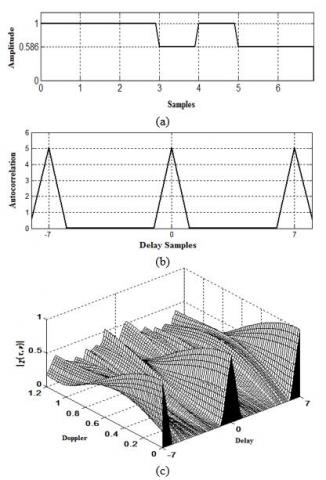Figure 4. (a) Amplitude of transmitted signal (b) PACF (c) PAF of synthesized binary sequence N=7. S7=[1 1 1 -0.586 1 -0.586 -0.586]

Table 2. Sequences with perfect periodic auto and cross-correlation properties (a=0.586 and ā=-0.586)

 Sequence Length (N) Efficiency (η) (%) Synthesized sequences 28 72 S(1)= [ +  +   +   ā   +   ā    ā   +   +   +   ā   +   ā   ā   +   +   +  ā   +   ā   ā   +   +   +   ā  +   ā   ā] S(2) = [+    -     +    a   +   a   ā   -   +   -   ā   -  ā   a   +   -   +  a  +   a   ā  -   +   -   ā  -   ā   a] S(3) = [+  +   -   a   +   ā    a   -   +   +  a   -   ā   ā   -   -   +   ā   -   a   ā    +   -   -   ā   +   a   a] S(4)= [+  -   -   ā   +   a   a    +   +   -   a   +  ā   a   -   +   +  a   -   ā   ā   -    -   +   ā   -   a   ā ]

To demonstrate the perfect periodic auto and cross correlation properties, two sequences N1=7 of odd length and Walsh Hardmard matrix of N2=4 are considered. It is easy to see that GCD(7,4)=1 (greatest common divisor of 7 and 4 is 1), then the resulting synthesized sequences will have the length N=7×4=28.

A set of 4 synthesized sequences can be constructed by using this method. However, from Eq. (13) it can be observed that the sequence 3 and sequence 4 of Walsh Hadamard matrix, row 3 is one bit shifted version of row 4. When we take the product of the synthesized binary sequence with row 3 or row 4 of Hdamard matrix, the resulting sequences will have same periodic property (as explained in sec. 2.2). Hence, out of four sequences only three sequences will demonstrate perfect cross-correlation properties. Additionally, the resulting sequences have the same efficiency as the original signal that is N1 because the efficiency of the Hadamard sequence is 1 (i.e. 100%). By using “+” and “-” symbols for “1” and “1” and “a” and “ā” for 0.586 and ˗0.586 respectively, a set of four sequences is presented in Table 2. From Table 2, the sequences s(3) and s(4) are:

s(3) = [+ + - a +  ā  a -  +  + a - ā  ā -  -  +  ā -  a  ā  +  - - ā + a  a]

s(4) = [+ -  -  ā  +  a  a  + + -  a  +  ā  a -  + + a -   ā   ā -  -  +  ā - a  ā ].

The above two sequences clearly show that these are the shifted versions of each other. Hence, the periodic autocorrelation and cross-correlation property of these two sequences are similar. Therefore, only a set of three sequences (i.e. either s(1) s(2) s(3)  or s(1) s(2) s(4)) will exhibit perfect cross-correlation property. The perfect cross-correlation property of s(1) s(2) and s(3) is shown in Figure 6. If the large number of sequences in a set are required, we must consider the higher order of Walsh Hadamard matrix. Figure 5 shows the ideal ACF property of s(1) s(2) s(3).

## 5.png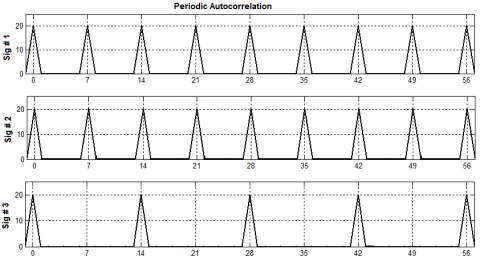Figure 5. Periodic autocorrelation of sequences s(1) s(2) and s(3) shown in Table 2

## 6.png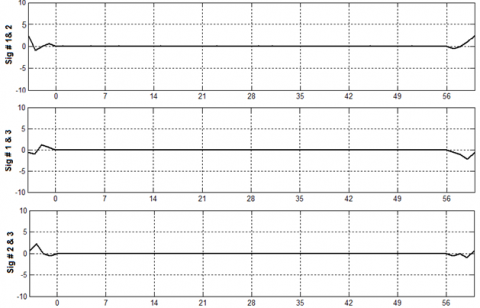Figure 6. Periodic cross-correlation of sequences s(1) s(2) and s(3) shown in Table 2

## 71.png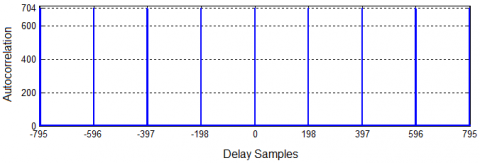(a) Periodic autocorrelation of signal s(1) N=796

## 72.png(b) Periodic autocorrelation of signal s(2) N=796

## 73.png(c) Periodic cross-correlation of signals s(1) and  s(2)

Figure 7. Periodic auto and cross-correlations of two signals for N= 796 (199x4)

It is evident from the Figures 5 and 6 that the proposed sequences have perfect autocorrelation and cross correlation properties. Additionally, Figure 7 establishes the perfect periodic auto ans cross correlation properties of higher length N=796, which is the product of two sequences (N1= 199, N2=4). It is clearly observed that the zero correlation zone in autocorrelation is (N1-1 =198) and in cross-correlation graph ZCZ extends from -796 to +796. The impulse like autocorrelation function in Figures 7(a) and 7(b) shows the range resolution capability of these sequences. Performance of synthesized sequences in presence of Doppler shift is examined in section 4.1 with the help of periodic cross ambiguity function.

4.1 Periodic cross ambiguity function

When the transmitted signal reflects back from a moving target, that results phase shift in received signal. This shift in received signal is corresponding to a Doppler shift. The main outcome of Doppler shift is that the autocorrelation peak will be reduced and also changes the sidelobe values in ambiguity function. To analyze the performance of periodic signals, Levanon [1, 9] introduced the concept of periodic ambiguity function. The PAF, of any single sequence can be represented by (17):

$\left|\chi_{T}(\tau, v)\right|_{\text {single}}=$

$|\frac{1}{T} \int_{0}^{T} s(t+\tau / 2) s^{*}(t-\tau / 2) \exp (j 2 \pi v t) d t \mid$     (17)

When PAF of two different signals s(1) and s(2)  is considered that is referred as periodic cross-ambiguity function and can be achieved by replacing $\left|\chi_{T}(\tau, v)\right|$ in Eq. (10) with $\left|\chi_{T}(\tau, v)\right|_{\text {pair}}$ which is given in (18).where, T is one period of the signal.

$\left|\chi_{T}(\tau, v)\right|_{\text {pair}}=$

$|\frac{1}{T} \int_{0}^{T} s^{(1)}(t+\tau / 2) s^{(2)^{*}}(t-\tau / 2) \exp (j 2 \pi v t) d t|$     (18)

Figure 8 is showing the un-normalized periodic cross-ambiguity function of two sequence s(1) and s(2). This figure concludes that the cross-correlation is perfect for zero Doppler shift. When Doppler shift in not zero perfect cross -correlation property does not hold. From the figure, when 28υtb (Doppler axis) is equal to 0.5 the maximum peak is 0.16 compared to the autocorrelation peak height of 1.00. This relationship shows that 28 vtb=0.5 or υ=0.018/tb, where v is doppler shift and tbis the duration of one bit of the sequence. Let the value of tb=0.1 microsecond, then the value of υ=0.18 MHz will cause such high cross-correlation peaks. It concludes that the periodic cross-ambiguity function of two signals exhibit such high cross-correlation peaks only when the carrier frequencies of the two signals are different by Δf=0.18 MHz. If two radars are operating in 10 GHz, such high peaks will appear when a target is moving with a speed of 2700 meters/sec.

## 8.png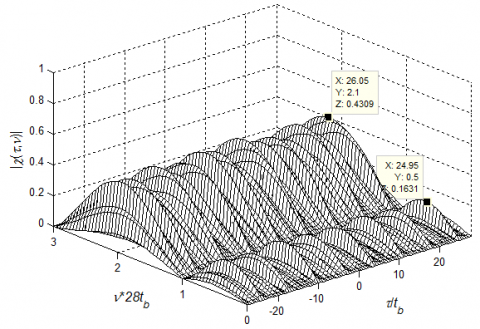Figure 8. Periodic cross-ambiguity function of sequences s(1) and s(2) of length N=28 (shown in Table 2)

5. Results and Discussions

Design of synthesized sequences, as applicable to multi-radar network and dense target environment, particularly when radars are employing coherent pulse train or CW waveforms have been presented in this paper. Two major issues are addressed here. (i) perfect periodic synthesized binary sequences with variable amplitude and higher energy efficiency are designed to achieve zero sidelobes in zero correlation zone so that even a small target present near the large target can be detected. (ii) to avoid the interference of multiple waveforms in applications such as RSN, MIMO radar or CDMA communications, it is desired to make sidelobes as low as possible [16-22]. The perfect periodic autocorrelation property of synthesized sequences are shown in Figures 4(b), 5, 7(a) and 7(b). Perfect periodic cross-correlation property of waveforms explained in section 4 is verified in Figures 6 and 7(c). The periodic cross-ambiguity function of the two sequences s(1) and s(2)  is shown in Figure 8 for length n=28, which represent the doppler shift property of synthesized sequences shown in Table 2.

For the detection performance evaluation of a radar system, the common parameters are probability of detection (PD), probability of false alarm (Pf) and probability of miss detection (PM). The relationship between PM and PD is given by PM=(1− PD). Therefore, only two parameters that is PD and Pf are sufficient to reveal the performance of designed sequences. To demonstrate the detection performance of proposed sequences, three types of sequences are considered. These three sequences are: proposed code which are referred as synthesized sequence, optimized PZCZSPS sequence, which perform promising in multi-radar network [5, 15, 16] and Gold code or gold sequence . Gold codes are well-known binary sequences which have good aperiodic auto and cross correlation properties.

Detection performance of these three types of sequences are simulated in Figure 9 for three different values of Pf considering single radar system. Figure 9(a) shows the graph of PD Vs SNR while Pf is kept 10-2. To achieve the PD = 0.9, the proposed synthesized code requires 1.0 dB of SNR. To achieve the same value of probability of detection using optimized PZCZPS and Gold code the SNR values are about 2.5 dB and 3.0 dB respectively. Similarly, in Figure 9(b), the value of Pf=10-3. Probability of detection PD=0.9 can be achieved with SNR value of 1.5 dB by using synthesized sequence whereas for optimized PZCZPS and Gold code SNR values are nearly 3.25 dB and 3.5 dB respectively to achieve the same PD. In Figure 9(c), synthesized sequence needs 2.0 dB of SNR to achieve PD=0.9. For optimized PZCZPS and Gold code the SNR values are approximately 3.4 dB and 4.2 dB respectively to achieve same value of PD. In Figures 9(a)-(c), detection performance of synthesized sequence of length 28 is compared with optimized PZCZPS of length 28 and Gold sequence of length 31. For each SNR value, Monte-Carlo simulation has been run 104 times. Analysis shown in Figures 9(a)-(c) establishes that the detection performance of synthesized sequences is significantly better than the optimized PZCZPS sequences and Gold codes.

## 9a.png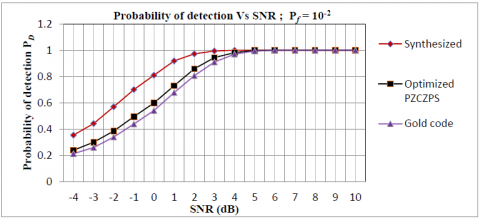(a) Pf =10-2

## 9b.png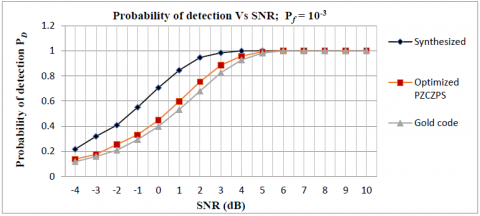(b) Pf=10-3

## 9c.png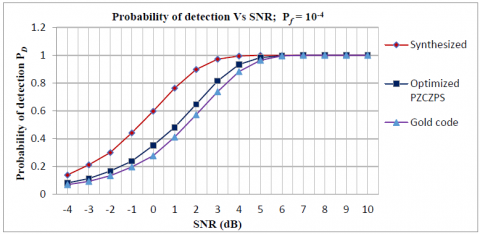(c) Pf =10-4

Figure 9. Probability of detection Vs SNR for different values of Pf: (a) Pf=10-2 (b) Pf=10-3 (c) Pf=10-4

6. Conclusions

This paper explores the design procedure of synthesized sequences with perfect periodic auto and cross-correlation properties. Sec. 3 describes the design of synthesized binary sequences with variable amplitude using M-sequences and Legendre sequences of odd lengths which have higher energy efficiency and perfect periodic autocorrelation properties. Construction procedure of proposed synthesized sequences having perfect periodic autocorrelation as well as cross correlation properties is explained in Sec. 4. Figures 5, 7(a) and 7(b) show the perfect periodic ACF of synthesized sequences, whereas Figures 6 and 7(c) are displaying the perfect periodic cross-correlation property within the zero-correlation zone. The periodic cross-ambiguity function shown in Figure 8 represents that the synthesized sequences are reasonably good even in higher Doppler shift. In any multi-radar scenario, when radars are employing coherent pulse train or CW waveforms, proposed sequences are predominantly suitable. The significant advantage of the proposed sequences is that the mutual cross-correlation values in zero correlation zone is very low. Additionally, impulse like autocorrelation function shown in Figures 7(a) and 7(b) displays the high range resolution capability of proposed sequences. Based on ideal correlation properties and higher energy efficiency, the proposed codes can be used in multiple radar systems, sharing the same frequency band for avoiding mutual interferences and enhancing detection and measurement performance of the radar systems.

Nomenclature

τ        time delay

υ       Doppler shift

η       energy efficiency

χ       ambiguity function

tB      duration of single bit

PD    probability of detection

Pf      probability of false alarm

PM    probability of miss detection

References

 Levanon, N., Mozeson, E. (2004). Radar Signals. Hoboken, NJ: Wiley.

 Luke, H.D. (1988). Sequences and arrays with perfect periodic correlation. IEEE Trans. on Aerospace and Electronic Systems, 24(3): 287-294. https://doi.org/10.1109/7.192096

 Saunders, W.K. (1990). CW and FM Radar. In M.I. Skolnik, Radar Hand Book, 2nd ed., Chap.14. New York, McGraw Hill.

 Levanon, N. (1993). CW alternatives to the coherent pulse train signals and processors. IEEE Trans. on Aerospace and Electronics Systems, 29(1): 250-254. https://doi.org/10.1109/7.249132

 Xu, L., Liang, Q. (2011). Radar sensor network using a set of new ternary codes: Theory and application. IEEE Sensors Journal, 11(2): 439-450. https://doi.org/10.1109/JSEN.2010.2060323

 Liang, Q. (2006). Waveform design and diversity in radar sensor networks: theoretical analysis and application to automatic target recognition. IEEE Sensor and Ad Hoc Communications and Networks Conference, 2(28): 684-689. https://doi.org/10.1109/SAHCN.2006.288531

 Deng, H. (2004). Polyphase code design for orthogonal netted radar systems. IEEE Transactions on Signal Processing, 52(11): 3126-3135. https://doi.org/10.1109/TSP.2004.836530

 Nenashev, A.V. (1986). Properties of the periodic ambiguity function for polyphase quadratic sequences. Radio Electronics and Communications Systems, 29(4): 113-114. https://doi.org/10.1109/7.303781

 Levanon, N., Freedman, A. (1992). Periodic ambiguity function of CW signals with perfect periodic autocorrelation. IEEE Trans. on Aerospace and Electronic Systems, 28(2): 387-395. https://doi.org/10.1109/7.144564

 Freedman, A., Levanon, N. (1994). Properties of the periodic ambiguity function. IEEE Transactions on Aerospace and Electronic Systems, 30(3): 938-941. https://doi.org/10.1109/7.303781

 Getz, B., Levanon, N. (1995). Weight effects on the periodic ambiguity function. IEEE Trans. on Aerospace and Electronic Systems, 31(1): 182-193. https://doi.org/10.1109/7.366302

 Ipatov, V.P. (1992). Periodic discrete signals with optimal correlation properties. Moskva: "Radio i Sviaz".

 Levanon, N., Cohen, I., Arbel, N., Zadok, A. (2016). Non-coherent pulse compression- aperiodic and periodic waveforms. IET Radar Sonar and Navigation, 10(1): 216-224. http://dx.doi.org/10.1049/iet-rsn.2015.0046

 Jahangir, K.K., Ali, J.H. (2016). A novel non-coherent radar pulse compression technique based on periodic m-sequences. Aerospace Science and Technology, 53: 188-193. https://doi.org/10.1016/j.ast.2016.03.021

 Xu, L., Liang, Q. (2010). Optimized punctured ZCZ sequence-pair set: Design, analysis, and application to radar system. Journal of Wireless Com Network, 254837. https://doi.org/10.1155/2010/254837

 Xu, L., Liang, Q. (2012). Zero correlation zone sequence pair sets for MIMO radar. IEEE Trans. on Aerospace and Electronic Systems, 48(3): 2100-2113. https://doi.org/10.1109/TAES.2012.6237581

 Gold, R. (1967). Optimal binary sequences for spread spectrum multiplexing (Corresp.). IEEE Transactions on Information Theory, 13(4): 619-621. https://doi.org/10.1109/TIT.1967.1054048

 Liu, Y., Chen, C., Su, Y.T. (2013). New constructions of zero-correlation zone sequences. IEEE Transactions on Information Theory, 59(8): 4994-5007. https://doi.org/10.1109/TIT.2013.2253831

 Feng, L., Wang, J., Hu, R.Q. (2015). New design of optical zero correlation zone codes in quasi-synchronous VLC CDMA systems. EURASIP Journal on Wireless Communications and Networking, 120. https://doi.org/10.1186/S13638-015-0360-z

 Fassi, B., Ali, D., Taleb-Ahmed, A. (2014). Ternary zero correlation zone sequence sets for asynchronous DS-CDMA. Communications and Network, 6: 209-217. https://doi.org/10.4236/cn.2014.64023

 Lan, X., Zhang, M., Li, J.X. (2019). OFDM chirp waveform design based on subchirp bandwidth overlap and segmented transmitting for low correlation interference in MIMO radar. Sensors (Basel), 19(12): 2696. https://doi.org/10.3390/s19122696

 Liang, T., Zhu, Y., Qiang, F. (2017). Designing waveform sets with good correlation and stop band properties for MIMO radar via the gradient-based method. Sensors, 17(5): 999. https://doi.org/10.3390/s17050999